library(MetaculR)
Metaculus_response_login <- MetaculR_login()

# Improving current predictions

## Aggregate community’s forecasts

Other Metaculus predictions provide a wealth of insight about a question, MetaculR_myPredictions() quickly puts together a number of summary statistics.

questions_myPredictions <- MetaculR_myPredictions(guessed_by = Metaculus_response_login$Metaculus_user_id) MetaculR_aggregated_forecasts(questions_myPredictions, Metaculus_id = 8997) %>% knitr::kable() ID community_q2 community_ave community_q2_unweighted community_ave_unweighted community_mean_logodds community_mean_logodds_extremized_baseline 8997 0.72 0.7213863 0.78 0.7737288 0.7291571 0.8467761 ## Generate probabilistic consensus Sometimes you find external forecasts relevant to your question, but how to combine them? Using some work from McAndrew & Reich (2020), this provides a quick way to combine forecasts. As an example, this question asked, What will be the 13-month mean sunspot number for the year of Solar Cycle 25’s maximum? and Table 1 of “The Next Solar Cycle” published a number of early forecasts. Here you see that we combine forecasts using normal distributions as well as min-mode-max as well as 20p-80p forecasts. Additionally, we gave Pishkalo a weight of 0.2 (while the others default to 1) so that their very narrow range doesn’t dominate our consensus. forecasts <- list(list(range = c(0, 250), resolution = 1), list(source = "Pishkalo", dist = "Norm", params = c("mu", "sd"), values = c(116, 12), weight = 0.2), list(source = "Miao", dist = "Norm", params = c("mu", "sd"), values = c(121.5, 32.9)), list(source = "Labonville", dist = "TPD", params = c("min", "mode", "max"), values = c(89-14, 89, 89+29)), list(source = "NOAA", dist = "PCT", params = c(0.2, 0.8), values = c(95, 130)), list(source = "Han", dist = "Norm", params = c("mu", "sd"), values = c(228, 40.5)), list(source = "Dani", dist = "Norm", params = c("mu", "sd"), values = c(159, 22.3)), list(source = "Li", dist = "Norm", params = c("mu", "sd"), values = c(168, 6.3)), list(source = "Singh", dist = "Norm", params = c("mu", "sd"), values = c(89, 9))) prob_consensus <- MetaculR_probabilistic_consensus( f = forecasts) prob_consensus$summary %>%
knitr::kable()
source q10 q25 q50 mu q75 q90
10% Pishkalo 101 108 116 116.0000 124 131
10%1 Miao 79 99 122 121.5075 144 164
10%2 Labonville 83 87 93 94.0000 100 107
10%3 NOAA 47 97 112 114.6000 127 190
10%4 Han 169 191 213 208.5179 231 242
10%5 Dani 130 144 159 158.9980 174 188
10%6 Li 160 164 168 168.0000 172 176
10%7 Singh 77 83 89 89.0000 95 101
10%8 agg 84 95 128 135.8088 170 204

prob_consensus$pdf %>% tidyr::pivot_longer(cols = c(-x, -agg)) %>% ggplot2::ggplot() + ggplot2::geom_line(ggplot2::aes(x = x, y = value, color = name), alpha = 0.2) + ggplot2::geom_line(ggplot2::aes(x = x, y = agg * (ncol(prob_consensus$pdf) - 2)), # 8 forecasters, make aggregated density appear larger in plot
linetype = "dotted") +
ggplot2::geom_segment(ggplot2::aes(x = prob_consensus$summary$q50[nrow(prob_consensus$summary)], xend = prob_consensus$summary$q50[nrow(prob_consensus$summary)],
y = 0,
yend = prob_consensus$pdf$agg[which(prob_consensus$pdf$x == prob_consensus$summary$q50[nrow(prob_consensus$summary)])] * (ncol(prob_consensus$pdf) - 2)),
linetype = "dashed") +
ggplot2::theme_classic() +
ggplot2::labs(y = "density")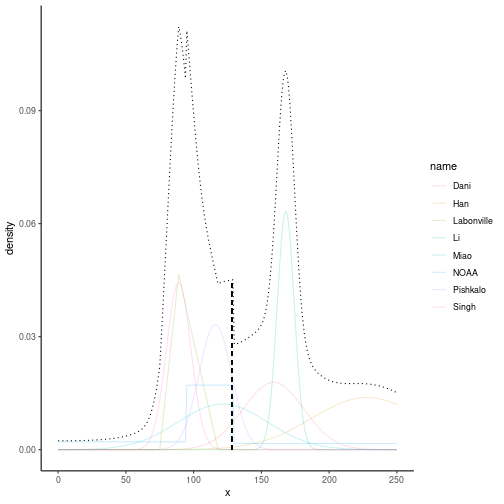plot of chunk unnamed-chunk-8

# Scoring

## Tournament Scoring

See your cumulative mean relative log score on questions where you have made predictions. If the question is unresolved (or ambiguously resolved), see both “Yes” and “No” possibilities, otherwise see only the resolution possibility.

MetaculR_plot(questions_myPredictions, 8997, tournament = TRUE)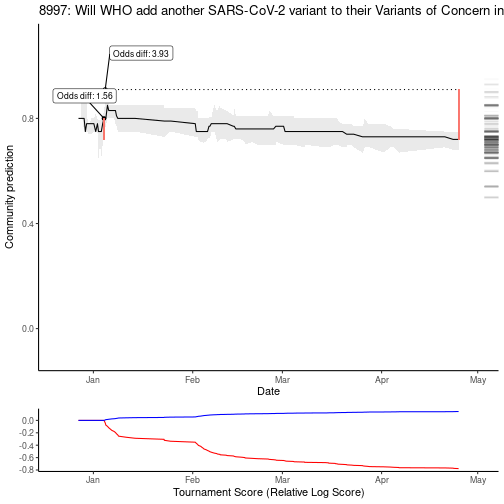plot of chunk unnamed-chunk-9

Watch your tournament score rise and fall over time.

MetaculR_plot(questions_myPredictions, 9939, tournament = TRUE)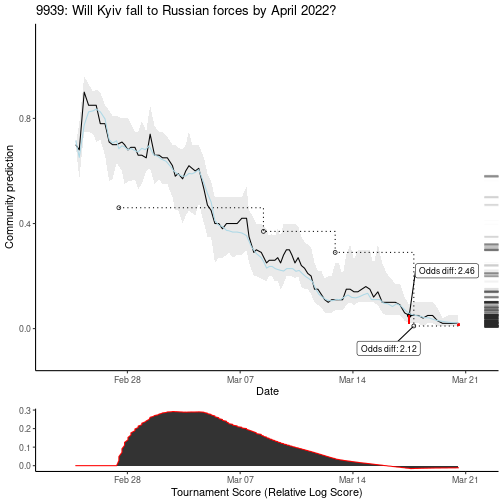plot of chunk unnamed-chunk-10

## Score Predictions (Equivalent Evidentiary Bins)

What if question bins for histograms, calibration plots, etc. were not 10 percentage points each, but were based on logodds? Use the thresholds argument of MetaculR_brier().

# questions_myPredictions_resolved <- MetaculR_myPredictions_Resolved(guessed_by = Metaculus_response_login$Metaculus_user_id) brier_me <- MetaculR_brier(questions_myPredictions, thresholds = c(0, exp(seq(from = -log(99), to = log(99), by = log(99) / 5)) / (exp(seq(from = -log(99), to = log(99), by = log(99) / 5)) + 1), 1)) #> Warning in x$results$possibilities$type == "binary" & !is.na(x$results$resolution) & : longer object length is not a multiple
#> of shorter object length

#> Warning in x$results$possibilities$type == "binary" & !is.na(x$results$resolution) & : longer object length is not a multiple #> of shorter object length #> Warning in x$results$possibilities$type == "binary" & !is.na(x$results$resolution) & : longer object length is not a multiple
#> of shorter object length

#> Warning in x$results$possibilities$type == "binary" & !is.na(x$results$resolution) & : longer object length is not a multiple #> of shorter object length #> Warning in x$results$possibilities$type == "binary" & !is.na(x$results$resolution) & : longer object length is not a multiple
#> of shorter object length

#> Warning in x$results$possibilities$type == "binary" & !is.na(x$results$resolution) & : longer object length is not a multiple #> of shorter object length #> Warning in x$results$possibilities$type == "binary" & !is.na(x$results$resolution) & : longer object length is not a multiple
#> of shorter object length

#> Warning in x$results$possibilities$type == "binary" & !is.na(x$results$resolution) & : longer object length is not a multiple #> of shorter object length brier_me$brier_df  %>%
ggplot2::ggplot() +
ggplot2::geom_col(
ggplot2::aes(x = name,
y = value,
fill = ID),
position = "dodge2"
) +
ggplot2::geom_text(
ggplot2::aes(x = name,
y = value,
label = round(value, 3)),
size = 2,
position = ggplot2::position_dodge2(width = 1), #"dodge2",
vjust = 0.5,
hjust = -0.25,
angle = 90) +
ggplot2::theme_classic() +
ggplot2::labs(x = "Statistic",
y = "Value") +
ggplot2::coord_cartesian(ylim = c(-1, 1)) +
ggplot2::theme(axis.text.x = ggplot2::element_text(angle = 45, vjust = 1, hjust = 1))
#> Warning: Removed 17 rows containing missing values (geom_col).
#> Warning: Removed 17 rows containing missing values (geom_text).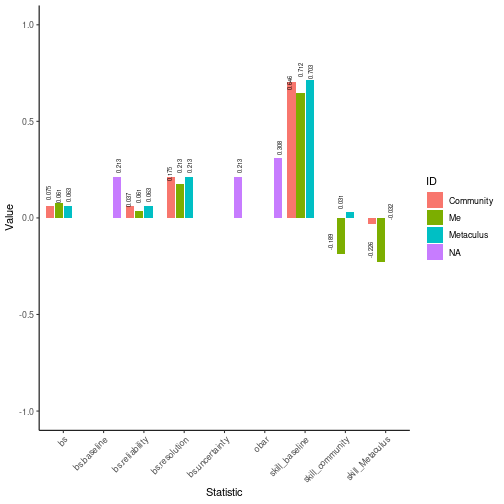plot of chunk unnamed-chunk-13

brier_me$brier_bins_df %>% ggplot2::ggplot() + ggplot2::geom_col( ggplot2::aes(x = centers, y = freqs, fill = ID), position = ggplot2::position_dodge2(width = 0.1, preserve = "single") ) + ggplot2::geom_text( ggplot2::aes(x = centers, y = freqs, label = round(freqs, 3)), size = 2, position = ggplot2::position_dodge2(width = 0.05), #"dodge2", # vjust = 0.5, hjust = -0.25, angle = 90) + ggplot2::theme_classic() + ggplot2::coord_cartesian(ylim = c(0, 1))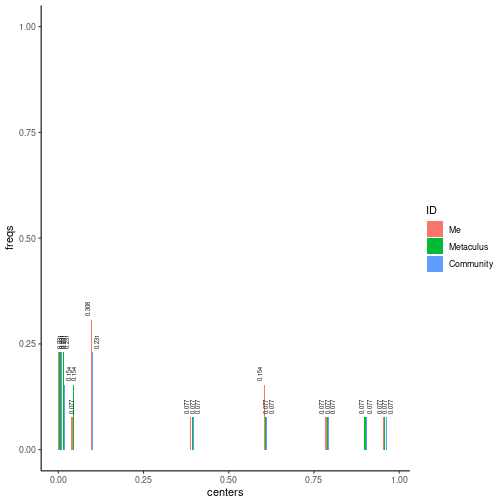plot of chunk unnamed-chunk-14 #### Calibration (Equivalent Evidentiary Bins) brier_me$brier_bins_df %>%
ggplot2::ggplot() +
ggplot2::geom_pointrange(
ggplot2::aes(x = centers,
y = obars,
ymin = ci_low,
ymax = ci_high,
color = ID),
position = ggplot2::position_dodge2(width = 0.02)
) +
ggplot2::geom_line(
ggplot2::aes(x = centers,
y = ideal)
) +
ggplot2::geom_text(
ggplot2::aes(x = centers,
y = obars,
label = format(round(obars, 3), nsmall = 3)),
size = 2,
position = ggplot2::position_dodge2(width = 0.1), #"dodge2",
vjust = -0.5,
hjust = -0.25,
angle = 90) +
ggplot2::theme_classic() +
ggplot2::coord_cartesian(ylim = c(0, max(brier_me$brier_bins_df$obars) * 1.1))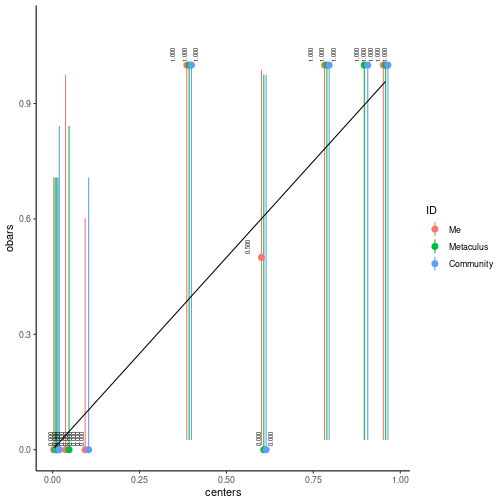plot of chunk unnamed-chunk-15